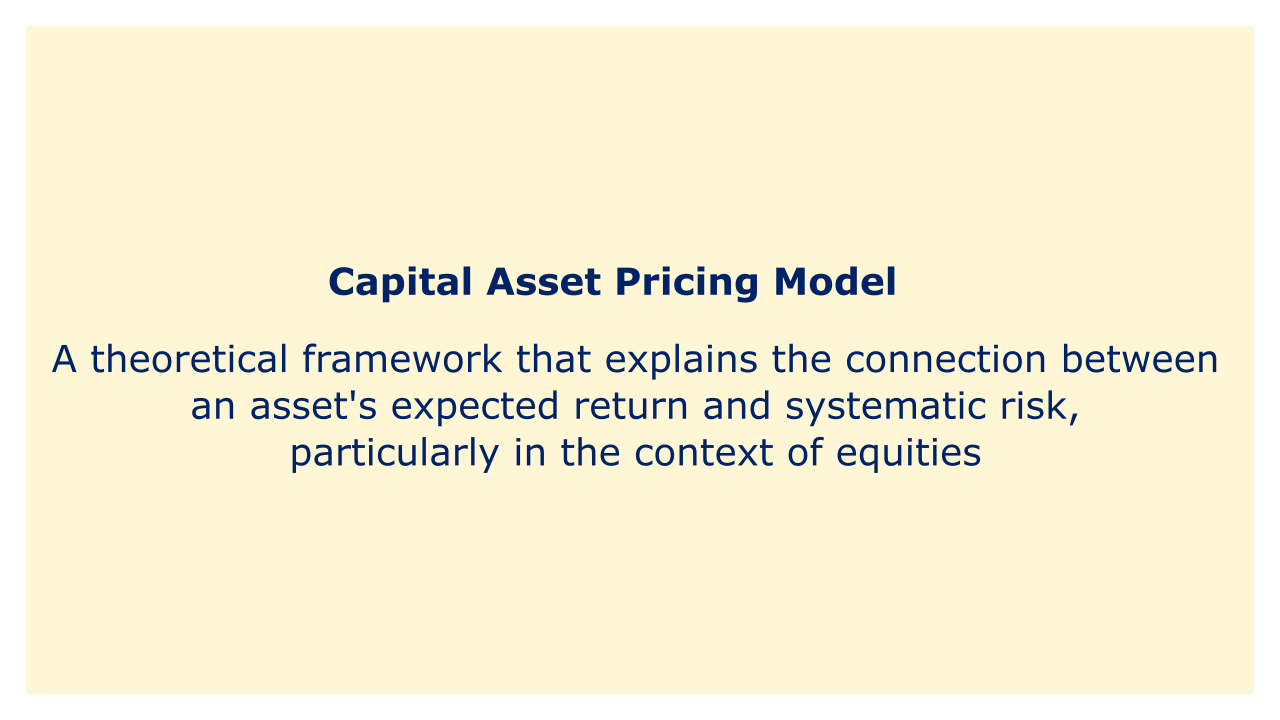# Capital Asset Pricing ModelImage: Moneybestpal.com

### The capital asset pricing model (CAPM) is a theoretical framework that explains the connection between an asset's expected return and systematic risk, particularly in the context of equities.

The model makes the assumption that investors are logical and risk-averse and that they can diversify away from the unsystematic risk of their portfolio by holding the market portfolio, which is a collection of all hazardous assets in the market combined and weighted according to their market prices.

According to the CAPM, an asset's expected return is equal to the risk-free rate plus a risk premium whose value is determined by the asset's beta, a metric of that asset's susceptibility to market risk. The market risk premium—the difference between the expected return of the market portfolio and the risk-free rate—and the beta together make up the risk premium. The CAPM can be expressed by the following formula:

Expected return = Risk-free rate + (Beta x Market risk premium)

The CAPM can be used to calculate the cost of capital for a project or business as well as to assess the performance of an asset or portfolio and estimate the required rate of return for an asset. The CAPM does, however, have certain drawbacks, including the difficulty in quantifying the market portfolio and beta, the model's irrational assumptions, and the difficulties in verifying the model empirically.
Tags